2022-07-19
###### Understanding Deep U-Nets for Semantic Segmentation: A salt identification case study with Keras
2022-07-19

Transposed Convolutions is a revolutionary concept for applications like image segmentation, super-resolution, etc but sometimes it becomes a little trickier to understand. In this post, I will try to demystify the concept and make it easier to understand.

## Introduction

Computer Vision Domain is going through a transition phase since gaining the popularity of Convolutional Neural Networks(CNN). The revolution started with Alexnet winning the ImageNet challenge in 2012 and since then CNNs have ruled the domain in Image Classification, Object Detection, Image Segmentation, and many other image/videos related tasks.

The Convolution operation reduces the spatial dimensions as we go deeper down the network and creates an abstract representation of the input image. This feature of CNNs is very useful for tasks like image classification where you just have to predict whether a particular object is present in the input image or not. But this feature might cause problems for tasks like Object Localization, and Segmentation where the spatial dimensions of the object in the original image are necessary to predict the output bounding box or segment the object.

To fix this problem various techniques are used such as fully convolutional neural networks where we preserve the input dimensions using ‘same’ padding. Though this technique solves the problem to a great extent, it also increases the computation cost as now the convolution operation has to be applied to original input dimensions throughout the network.

Another approach used for image segmentation is dividing the network into two parts i.e A Downsampling network and then an Upsampling network.
In the Downsampling network, simple CNN architectures are used and abstract representations of the input image are produced.
In the Upsampling network, the abstract image representations are upsampled using various techniques to make their spatial dimensions equal to the input image. This kind of architecture is famously known as the Encoder-Decoder network.

## Upsampling Techniques

The Downsampling network is intuitive and well-known to all of us but very little is discussed about the various techniques used for Upsampling.

The most widely used techniques for upsampling in Encoder-Decoder Networks are:

1. Nearest Neighbors: In Nearest Neighbors, as the name suggests we take an input pixel value and copy it to the K-Nearest Neighbors where K depends on the expected output.

2. Bi-Linear Interpolation: In Bi-Linear Interpolation, we take the 4 nearest pixel values of the input pixel and perform a weighted average based on the distance of the four nearest cells smoothing the output.

3. Bed Of Nails: In Bed of Nails, we copy the value of the input pixel at the corresponding position in the output image and fill in zeros in the remaining positions.

4. Max-Unpooling: The Max-Pooling layer in CNN takes the maximum among all the values in the kernel. To perform max-unpooling, first, the index of the maximum value is saved for every max-pooling layer during the encoding step. The saved index is then used during the Decoding step where the input pixel is mapped to the saved index, filling in zeros everywhere else.

All the above-mentioned techniques are predefined and do not depend on data, which makes them task-specific. They do not learn from data and hence are not a generalized technique.

## Transposed Convolutions

Transposed Convolutions are used to upsample the input feature map to a desired output feature map using some learnable parameters.
The basic operation that goes in a transposed convolution is explained below:
1. Consider a 2×2 encoded feature map that needs to be upsampled to a 3×3 feature map.

2. We take a kernel of size 2×2 with unit stride and zero padding.

3. Now we take the upper left element of the input feature map and multiply it with every element of the kernel.

4. Similarly, we do it for all the remaining elements of the input feature map.

5. As you can see, some of the elements of the resulting upsampled feature maps are overlapping. To solve this issue, we simply add the elements of the overlapping positions.

6. The resulting output will be the final upsampled feature map having the required spatial dimensions of 3×3.

Transposed convolution is also known as Deconvolution which is not appropriate as deconvolution implies removing the effect of convolution which we are not aiming to achieve. It is also known as upsampled convolution which is intuitive to the task it is used to perform, i.e upsample the input feature map. It is also referred to as fractionally strided convolution since a stride over the output is equivalent to a fractional stride over the input. For instance, a stride of 2 over the output is 1/2 stride over the input. Finally, it is also referred to as Backward strided convolution because a forward pass in a Transposed Convolution is equivalent to a backward pass of a normal convolution.

## Performing Transposed Convolution using Standard Convolution

### Standard Convolutional Layer

A standard convolutional layer on an input of size i*i is defined by the following two parameters.

• Padding (p): The number of zeros padded around the original input increasing the size to (i+2*p)x(i+2*p)
• Stride (s): The amount by which the kernel is shifted when sliding across the input image.

The figure below shows how a convolutional layer works as a two-step process.

In the first step, the input image is padded with zeros, while in the second step the kernel is placed on the padded input and slid across generating the output pixels as dot products of the kernel and the overlapped input region. The kernel is slid across the padded input by taking jumps of size defined by the stride. The convolutional layer usually does a down-sampling i.e. the spatial dimensions of the output are less than that of the input.

The animations below explain the working of convolutional layers for different values of stride and padding.

For a given size of the input (i), kernel (k), padding (p), and stride (s), the size of the output feature map (o) generated is given by

## Transposed Convolutional Layer

A transposed convolutional layer, on the other hand, is usually carried out for upsampling i.e. to generate an output feature map that has a spatial dimension greater than that of the input feature map. Just like the standard convolutional layer, the transposed convolutional layer is also defined by padding and stride. These values of padding and stride are the one that hypothetically was carried out on the output to generate the input. i.e. if you take the output, and carry out a standard convolution with stride and padding defined, it will generate the spatial dimension the same as that of the input.

Implementing a transposed convolutional layer can be better explained as a 4 step process

• Step 1: Calculate new parameters z and p’
• Step 2: Between each row and column of the input, insert z number of zeros. This increases the size of the input to (2*i-1)x(2*i-1)
• Step 3: Pad the modified input image with p’ number of zeros
• Step 4: Carry out standard convolution on the image generated from step 3 with a stride length of 1

The complete steps can be seen in the figure below.

The animations below explain the working of convolutional layers for different values of stride and padding.

For a given size of the input (i), kernel (k), padding (p), and stride (s), the size of the output feature map (o) generated is given by

## Difference between UpSampling2D and Conv2DTranspose in Keras

UpSampling2D is just a simple scaling up of the image by using the nearest neighbor or bilinear upsampling, so nothing smart. Its advantage is it’s cheap.

Conv2DTranspose is a convolution operation whose kernel is learned (just like a normal conv2d operation) while training your model. Using Conv2DTranspose will also upsample its input but the key difference is the model should learn what is the best upsampling for the job.

Link to nice visualization of transposed convolution: https://towardsdatascience.com/types-of-convolutions-in-deep-learning-717013397f4d

## Transposed Convolution in Keras

Transposed convolution is the opposite of convolution. In the convolutional layer, we use a special operation named cross-correlation (in machine learning, the operation is more often known as convolution, and thus the layers are named “Convolutional Layers”) to calculate the output values. This operation adds all the neighboring numbers in the input layer together, weighted by a convolution matrix (kernel). For example, in the image below, the output value 55 is calculated by the element-wise multiplication between the 3×3 part of the input layer and the 3×3 kernel, and sum all results together: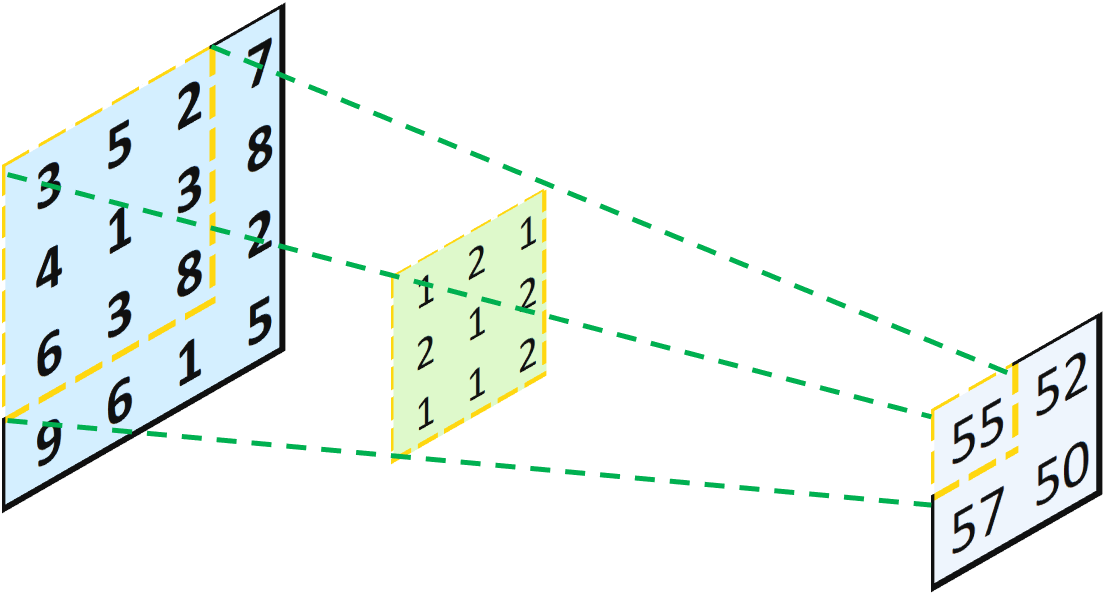One Convolution Operation With 3×3 Part of the Input Layer and the 3×3 Kernel

Without any padding, this operation transforms a 4×4 matrix into a 2×2 matrix. This looks like someone is casting the light from left to right, and projecting an object (the 4×4 matrix) through a hole (the 3×3 kernel), and yielding a smaller object (the 2×2 matrix). Now, our question is: what if we want to go backward from a 2×2 matrix to a 4×4 matrix? Well, the intuitive way is, that we just cast the light backward! Mathematically, instead of multiplying two 3×3 matrices, we can multiply each value in the input layer by the 3×3 kernel to yield a 3×3 matrix. Then, we just combine all of them together according to the initial positions in the input layer, and sum the overlapped values together: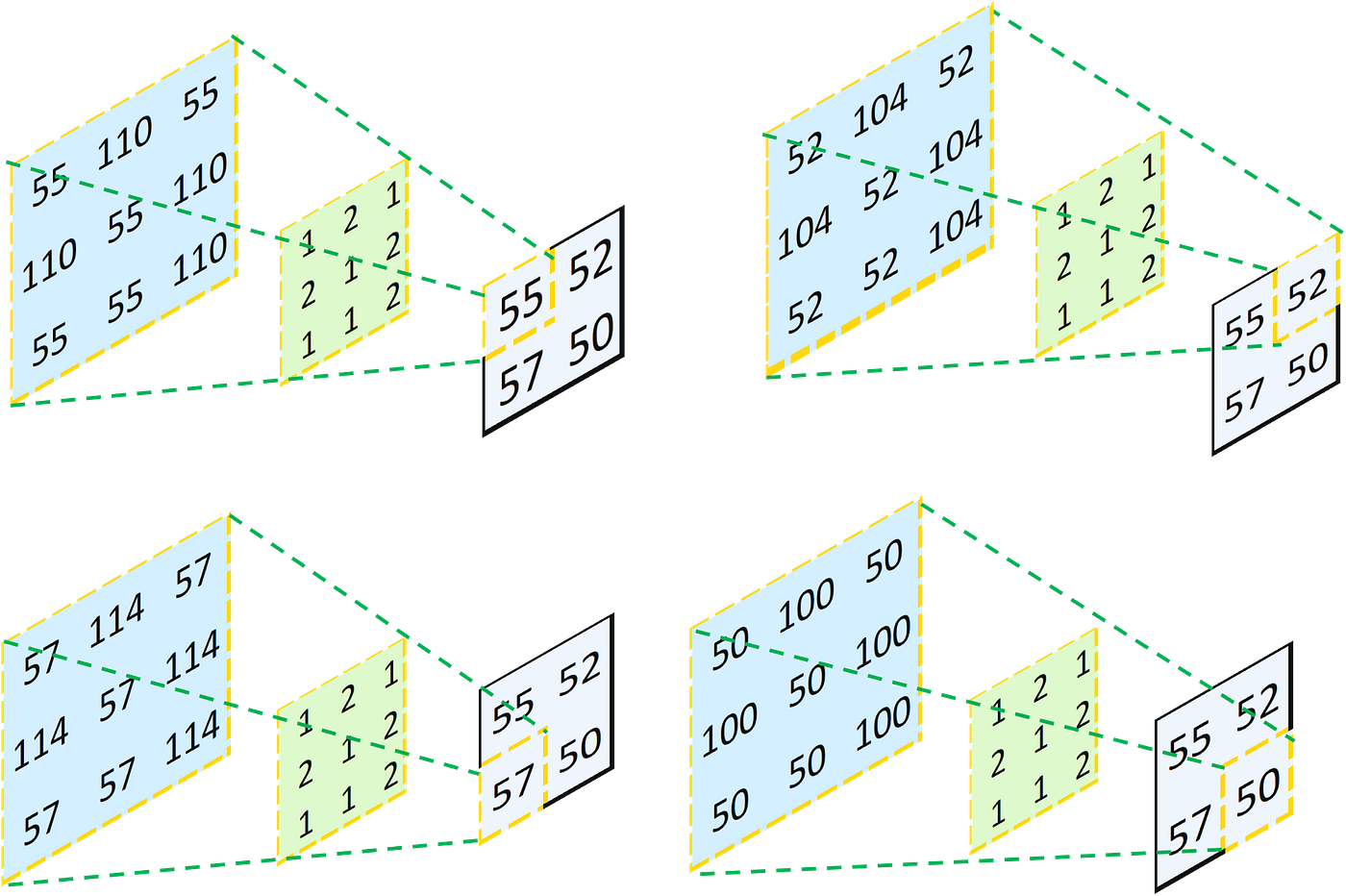Multiply Each Element in the Input Layer by Each Value in the Kernel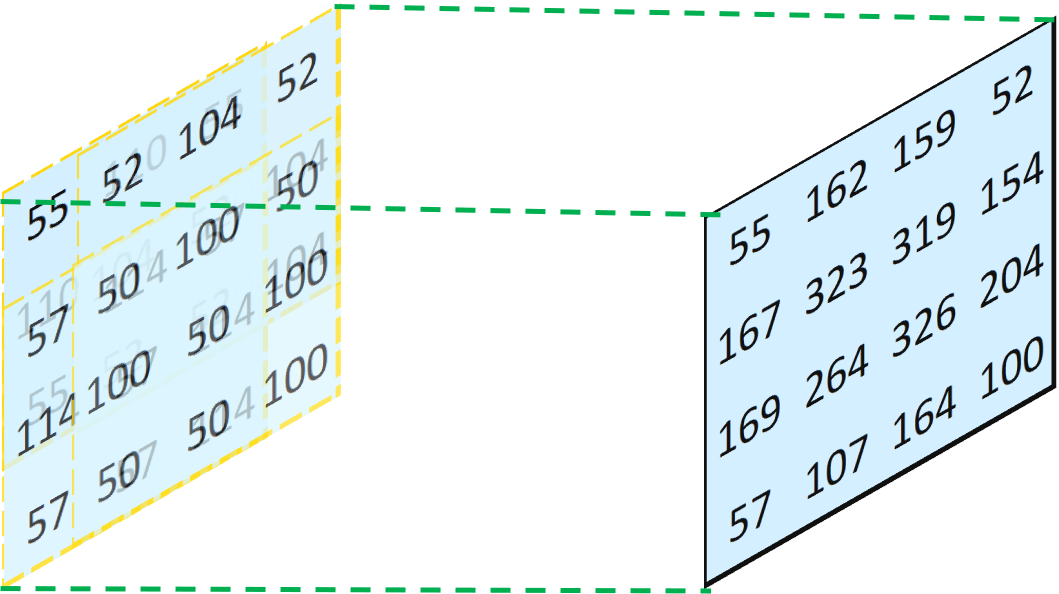Combine All Four Resulting Layers Together And Sum the Overlapped Values

In this way, it is always certain that the output of the transposed convolution operation can have exactly the same shape as the input of the previous convolution operation because we just did exactly the reverse. However, you may notice that the numbers are not restored. Therefore, a totally different kernel has to be used to restore the initial input matrix, and this kernel can be determined through training.

To demonstrate that my results are not just some random numbers, I build the convolutional neural networks using the conditions indicated above through Keras. As can be seen from the code below, the outputs are exactly the same.

```from tensorflow import keras
import numpy as np
X = np.array([[3, 5, 2, 7], [4, 1, 3, 8], [6, 3, 8, 2], [9, 6, 1, 5]])
X = X.reshape(1, 4, 4, 1)
model_Conv2D = keras.models.Sequential()
weights = [np.asarray([[[], [], []], [[], [], []], [[], [], []]]), np.asarray()]
model_Conv2D.set_weights(weights)
yhat = model_Conv2D.predict(X)
yhat.reshape(2, 2)
```
```X = yhat
model_Conv2D = keras.models.Sequential()
weights = [np.asarray([[[], [], []], [[], [], []], [[], [], []]]), np.asarray()]
model_Conv2D.set_weights(weights)
yhat = model_Conv2D.predict(X)
yhat.reshape(4, 4)
```

## Why “Transposed”?

Now that you may be wondering: hey, this looks just like a reversed convolution. Why is it named “transposed” convolution?

The reason it is a 4×16 matrix is that:

• 4 rows: in total, we can perform four convolutions by splitting a 4×4 input matrix into four 3×3 matrices;
• 16 columns: the input matrix will be transformed into a 16×1 vector. To perform the matrix multiplication, it has to be 16 columns.

In this way, we can directly perform the matrix multiplication to get an output layer. The reshaped output layer will be exactly the same as the one derived by the general convolution operation.

Now comes the most interesting part! When we perform transposed convolution operation, we just simply transpose the zero-padded convolution matrix and multiply it with the input vector (which was the output of the convolutional layer). In the picture below, the four colored vectors in the middle stage represent the intermediate step of matrix multiplication: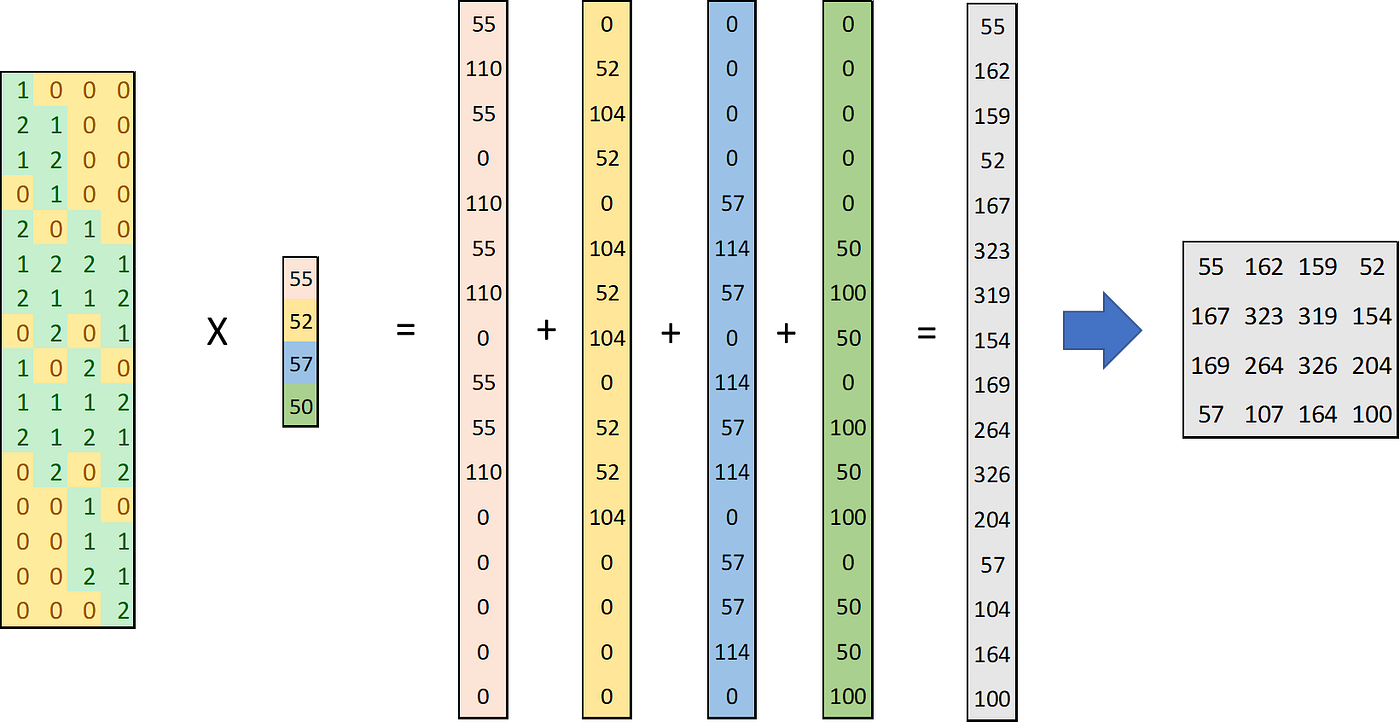Matrix Multiplication Between 16×4 Transposed Convolution Matrix and 4×1 Input Vector

If we rearrange the four vectors in the middle stage, we will get the four 4×4 matrices that have exactly the same numbers as the 3×3 matrices we obtained by multiplying the 3×3 kernel with each individual element in the input layer, with the extra slots filled by zeros. These four matrices can also be further combined to get the final 4×4 output matrix:

Thus, the operation is called “transposed” convolution because we performed exactly the same operation except that we transposed the convolution matrix!

## Parameters of Keras Conv2DTranspose

### 1. Kernel Size

In convolutions, the kernel size affects how many numbers in the input layer you “project” to form one number in the output layer. The larger the kernel size, the more numbers you use, and thus each number in the output layer is a broader representation of the input layer and carries more information from the input layer. But at the same time, using a larger kernel will give you an output with a smaller size. For example, a 4×4 input matrix with a 3×3 kernel will yield a 2×2 output matrix, while a 2×2 kernel will yield a 3×3 output matrix (if no padding is added):

In transposed convolutions, when the kernel size gets larger, we “disperse” every single number from the input layer to a broader area. Therefore, the larger the kernel size, the larger the output matrix (if no padding is added):

### 2. Strides

In convolutions, the strides parameter indicates how fast the kernel moves along the rows and columns on the input layer. If a stride is (1, 1), the kernel moves one row/column for each step; if a stride is (2, 2), the kernel moves two rows/columns for each step. As a result, the larger the strides, the faster you reach the end of the rows/columns, and therefore the smaller the output matrix (if no padding is added). Setting a larger stride can also decrease the repetitive use of the same numbers.

In transposed convolutions, the strides parameter indicates how fast the kernel moves on the output layer, as explained by the picture below. Notice that the kernel always moves only one number at a time on the input layer. Thus, the larger the strides, the larger the output matrix (if no padding).

In convolutions, we often want to maintain the shape of the input layers, and we do it through zero-padding. In Keras, the padding parameter can be one of two strings: “valid” or “same”. When padding is “valid”, it means no zero-padding is implemented. When padding is “same”, the input layer is padded in a way so that the output layer has a shape of the input shape divided by the stride. When the stride is equal to 1, the output shape is the same as the input shape.

In transposed convolutions, the padding parameter also can be the two strings: “valid” and “same”. However, since we expand the input layer in transposed convolutions, if choosing “valid”, the output shape will be larger than the input shape. If “same” is used, then the output shape is forced to become the input shape multiplied by the stride. If this output shape is smaller than the original output shape, then only the very middle part of the output is maintained.

An easier way to remember “valid” and “same” in both convolutions and transposed convolutions is:

• “valid”: no extra operation is performed. The output stays what it is meant to be.
• “same”: the output shape is the input shape divided by the stride (convolutions) or multiplied by the stride (transposed convolutions). When the stride is equal to 1, the output shape is always the same as the input shape.

## Build My Own Conv2D and Conv2DTransposed Layers From Scratch

Up to now, I have explained all the concepts about transposed convolutional layers and their important parameters. They may still be very abstract for you, and I totally understand you, because I also struggled a lot to understand how transposed convolutional layers work. But don’t worry, now we can get our hands dirty and build our own convolutional and transposed convolutional layers using the concepts we learned — this will definitely reveal the mystery of the transposed convolutional layers!

```from math import floor, ceil
def Conv2D(X, W, padding="valid", strides=(1, 1)):
# returns the output with the shape of (input shape)/(stride)
p_row = ceil(((X.shape/strides - 1) * strides + W.shape - X.shape)/2)
p_col = ceil(((X.shape/strides - 1) * strides + W.shape - X.shape)/2)
# returns the output without any padding
p_row = 0
p_col = 0
row_num = X.shape + 2 * p_row
col_num = X.shape + 2 * p_col
# Calculate the output
output = []
for i in range(0, X_padded.shape-W.shape+1, strides):
output.append([])
for j in range(0, X_padded.shape-W.shape+1, strides):
X_sub = X_padded[i:i+W.shape, j:j+W.shape] # Subset of X
output[-1].append(np.sum(X_sub * W))
return(np.array(output))
```

Let’s go through my home-made Conv2D layer:

• First, I defined the number of zero-padding I need to add. If the padding is “valid”, then I don’t need to add any padding. If the padding is “same”, I calculate the number of padding on each side of the input layer based on the formula:

Where:
– o is the output size
– s is the strides
– m is the kernel size
– is the input size
– p is the padding number on each side of the original input layer

This formula is derived from the formula for calculating the output shape:

With the output shape of o = n/s.

• Then, I padded the input by constructing a larger matrix filled with zeros and putting the original input in the middle.
• After that, I calculated the output using the convolution operation. The convolution operation is performed between the kernel W and the subset of input X_sub (which has the same shape as the kernel). The output indices i, j range from 0 to the last index that can fit in the kernel. For example, if X_padded has a shape of 4×4, and the kernel has a shape of 3×3, then the last index that can fit in the kernel is 1(the kernel can be fitted from index 1 to index 4). A graphical explanation of the process can be found below: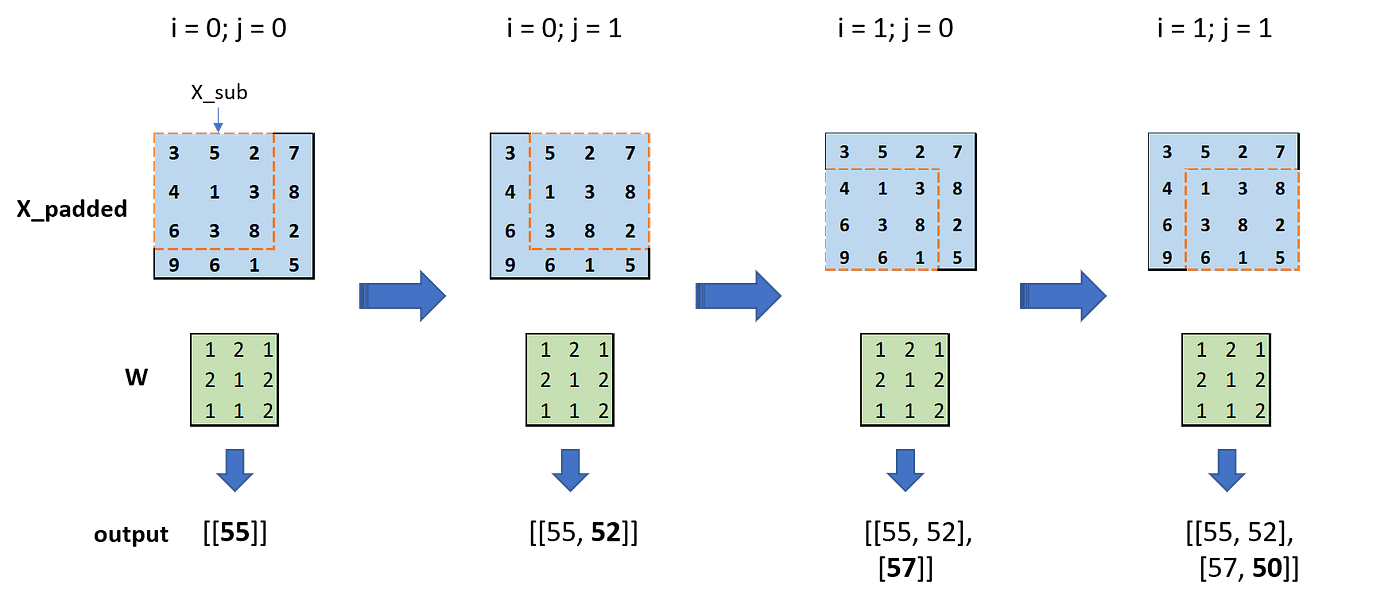Calculating the Output Using a 4×4 Input Matrix and a 3X3 Kernel. The Number Updated at Each Step in the Output is Bolded

I also compared the results using my Conv2D with Keras Conv2D. The results are the same!

```X = np.array([[3, 5, 2, 7], [4, 1, 3, 8], [6, 3, 8, 2], [9, 6, 1, 5]])
X_reshape = X.reshape(1, 4, 4, 1)
W = np.array([[1, 2, 1], [2, 1, 2], [1, 1, 2]])
my_output = Conv2D(X, W, padding="valid", strides=(1, 1))
print("My Conv2D: \n {}".format(my_output))
print("\n")
model_Conv2D = keras.models.Sequential()
weights = [np.asarray([[[], [], []], [[], [], []], [[], [], []]]), np.asarray()]
model_Conv2D.set_weights(weights)
keras_output = model_Conv2D.predict(X_reshape)
keras_output = keras_output.reshape(2, 2)
print("Keras Conv2D: \n {}".format(keras_output))
```
```X = np.array([[3, 5, 2, 7], [4, 1, 3, 8], [6, 3, 8, 2], [9, 6, 1, 5]])
X_reshape = X.reshape(1, 4, 4, 1)
W = np.array([[1, 2, 1], [2, 1, 2], [1, 1, 2]])
my_output = Conv2D(X, W, padding="same", strides=(1, 1))
print("My Conv2D: \n {}".format(my_output))
print("\n")
model_Conv2D = keras.models.Sequential()
weights = [np.asarray([[[], [], []], [[], [], []], [[], [], []]]), np.asarray()]
model_Conv2D.set_weights(weights)
keras_output = model_Conv2D.predict(X_reshape)
keras_output = keras_output.reshape(4, 4)
print("Keras Conv2D: \n {}".format(keras_output))
```

Now let’s build the transposed convolutional layer:

```from math import floor, ceil
def Conv2DTranspose(X, W, padding="valid", strides=(1, 1)):
# Define output shape before padding
row_num = (X.shape - 1) * strides + W.shape
col_num = (X.shape - 1) * strides + W.shape
output = np.zeros([row_num, col_num])
# Calculate the output
for i in range(0, X.shape):
i_prime = i * strides # Index in output
for j in range(0, X.shape):
j_prime = j * strides
# Insert values
for k_row in range(W.shape):
for k_col in range(W.shape):
output[i_prime+k_row, j_prime+k_col] += W[k_row, k_col] * X[i, j]
# returns the output with the shape of (input shape)*(stride)
p_left = floor((W.shape - strides)/2)
p_right = W.shape - strides - p_left
p_top = floor((W.shape - strides)/2)
p_bottom = W.shape - strides - p_left
# returns the output without any padding
p_left = 0
p_right = 0
p_top = 0
p_bottom = 0
```

Let’s break up the code:

• The output shape is firstly defined by the formula below:

If you compare the formula to calculate the output shape of Conv2D, you can notice that in Conv2DTranspose both the strides and the kernel size have the opposite effect on the output shape.

• To calculate the output, I use two pairs of indices: i, j move along the input, and i_prime, j_prime move along with the output. When i, j change, i_prime, j_prime change with step sizes of given strides. For example, if i changes from 0 to 1, and strides = (2, 2), then i_prime changes from 0 to 2. Each value in the input matrix is multiplied by all values in the kernel, and the results are recorded in the output matrix.
• Then, I defined the length of the padding. Again, if the padding is “valid”, I don’t need to modify anything. If the padding is “same”, then the output shape has to be the input shape multiplied by the stride. That is:

The padding has to convert the original output shape to the desired output shape:

And therefore an easy way to set the values of padding is:

• Lastly, the output is padded by only selecting the matrix in the middle that has the same shape as the input matrix.

A graphical explanation of the process of calculating the output is shown below:

Now we can verify our Conv2DTranspose function by comparing the results with Conv2DTranspose in Keras:

```X = np.array([[55, 52], [57,50]])
X_reshape = X.reshape(1, 2, 2, 1)
W = np.array([[1, 2], [2, 1]])
my_output = Conv2DTranspose(X, W, padding="valid", strides=(1, 1))
print("My Conv2D: \n {}".format(my_output))
print("\n")
model_Conv2D_Transpose = keras.models.Sequential()
weights = [np.asarray([[[], []], [[], []]]), np.asarray()]
model_Conv2D_Transpose.set_weights(weights)
keras_output = model_Conv2D_Transpose.predict(X_reshape)
keras_output = keras_output.reshape(3, 3)
print("Keras Conv2D: \n {}".format(keras_output))
```
```X = np.array([[55, 52], [57,50]])
X_reshape = X.reshape(1, 2, 2, 1)
W = np.array([[1, 2], [2, 1]])
my_output = Conv2DTranspose(X, W, padding="same", strides=(1, 1))
print("My Conv2D: \n {}".format(my_output))
print("\n")
model_Conv2D_Transpose = keras.models.Sequential()
weights = [np.asarray([[[], []], [[], []]]), np.asarray()]
model_Conv2D_Transpose.set_weights(weights)
keras_output = model_Conv2D_Transpose.predict(X_reshape)
keras_output = keras_output.reshape(2, 2)
print("Keras Conv2D: \n {}".format(keras_output))
```

The results are exactly the same!

## Problems with Transposed Convolutions

Transposed convolutions suffer from chequered board effects as shown below.

The main cause of this is uneven overlap at some parts of the image causing artifacts. This can be fixed or reduced by using kernel-size divisible by the stride, for e.g taking a kernel size of 2×2 or 4×4 when having a stride of 2.

## Applications of Transposed Convolution

1. Super-Resolution:

2. Semantic Segmentation:

## Conclusion

Transposed Convolutions are the backbone of modern segmentation and super-resolution algorithms. They provide the best and most generalized upsampling of abstract representations.

The table below summarizes the two convolutions, standard and transposed.

• The idea behind transposed convolution is to carry out trainable upsampling
• Transposed convolutions are standard convolutions but with a modified input feature map.
• The stride and padding do not correspond to the number of zeros added around the image and the amount of shift in the kernel when sliding it across the input, as they would in a standard convolution operation.

Resources:

https://towardsdatascience.com/transposed-convolution-demystified-84ca81b4baba

https://d2l.ai/chapter_computer-vision/transposed-conv.html

https://towardsdatascience.com/types-of-convolutions-in-deep-learning-717013397f4d